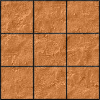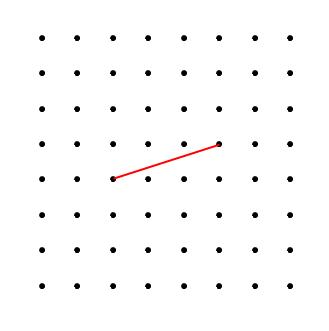#### You may also like### Paving the Way

A man paved a square courtyard and then decided that it was too small. He took up the tiles, bought 100 more and used them to pave another square courtyard. How many tiles did he use altogether?### Square Areas

Can you work out the area of the inner square and give an explanation of how you did it?### Chess

What would be the smallest number of moves needed to move a Knight from a chess set from one corner to the opposite corner of a 99 by 99 square board?

# Opposite Vertices

### Why do this problem?

Many students think they are very familiar with squares and rhombuses...
This problem requires a real appreciation of the properties of these quadrilaterals in order to make and justify some interesting generalisations.

Here is an article that describes some of the background thinking that informed the creation of this problem.

### Possible approach

These printable worksheets may be useful: Opposite Vertices - Squares
Opposite Vertices - Rhombuses
10mm Dotty Grid

You may be interested in our collection Dotty Grids - an Opportunity for Exploration, which offers a variety of starting points that can lead to geometric insights.

This problem requires students to draw tilted squares reliably. This interactivity might be helpful to demonstrate to students what a tilted square looks like. Students could play Square It until they can reliably spot tilted squares on a dotty grid.

A possible start which involves the minimum of teacher input is to display the line below and say:"Imagine we are drawing squares and rhombuses with vertices on the dots of a square grid. This line can be
• the side of at least one square,
• the diagonal of at least one square,
• the side of at least one rhombus,
• the diagonal of at least one rhombus.
Work out how many different squares and rhombuses can include this line, as either a side or a diagonal."

This leads on to the challenge "In a while, I am going to ask you to work out how many squares and rhombuses could be drawn using a different line. The challenge will be to answer without doing any drawing."

Alternatively, start by showing the image of Charlie's rubbed out squares, and give students some time to recreate the squares on dotty paper. Once they have finished, ask them to compare in pairs - have they always drawn the same square? Are there any other possibilities?

Bring the class together and share the techniques students were using to complete the squares.
"Draw any line you like on your dotty paper, and then try to complete a square using your line as one of the sides."
...
"Has anyone found a line that they can't use as a side of a square?" (If someone has found one, display it on the board and ask the class to help.)
"Do you think we can always draw a square using any line?"
Give students some time to discuss this and come up with justifications for their answer.

Next show the image of Alison's diagonals and give students time to recreate the squares.
Again, ask them to share approaches.
"Draw any line you like on your dotty paper, and then try to complete a square using your line as the diagonal."
This time, it can't always be done. If students are struggling to work out when it can and can't be done, suggest that they draw some squares and see what the diagonals have in common.

Then move on to rhombuses.
"Draw any line you like on your dotty paper, and then try to complete a rhombus using your line as one of the sides. How many different rhombuses can you draw using your line?"
Give students time to do this for a number of different lines of their own choosing, and then bring the class together to share their findings.

"When we worked on squares, there were some lines that we couldn't use as a diagonal. Will the same be true for rhombuses?"
Once again, give students time to explore.

Finally, bring the class together to share their ideas and justify their findings.

One technique for testing ideas at the end is to set a specific challenge, for example, to draw some lines and ask them to determine how many rhombuses could be drawn using each line as a diagonal.

### Key questions

Is there a method for completing a square given one of the sides?
Is there a method for completing a square given its diagonal?
Is there a quick way to determine whether a given line could be the side or diagonal of a square with points on the dots of a square grid?

Is there a method for completing a rhombus given one of the sides?
Is there a method for completing a rhombus given its diagonal?
Is there a quick way to determine whether a given line could be the side or diagonal of a rhombus with points on the dots of a square grid?

### Possible extension

Vector Journeys challenges students to explore similar relationships using vector algebra.

### Possible support

The interactivity in Square Coordinates helps students to visualise tilted squares.